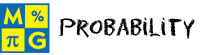# ProbabilityThis page lists the Learning Objectives for all lessons in Unit 6.

#### Introduction to Probability

The student will be able to:

• Define experiment, outcome, event, probability and equally likely.
• Restate the formula for finding the probability of an event.
• Determine the outcomes and probabilities for experiments.
• Interact with die rolls and spinners to help predict the outcome of experiments.
• Distinguish between an event and an outcome for an experiment.
• Recognize the difference between outcomes that are equally likely and not equally likely to occur.
• Apply probability concepts to complete five interactive exercises.

#### Certain and Impossible Events

The student will be able to:

• Define certain event, impossible event.
• Describe and list the contents of a standard deck of 52 playing cards.
• Examine the probabilities of experiments with certain outcomes.
• Examine the probabilities of experiments with impossible outcomes
• Evaluate interactive die rolls and spinners in relation to certain and impossible events.
• Explain the difference between certain and impossible events.
• Compute the probability of a certain event.
• Compute the probability of an impossible event.
• Apply concepts to complete five interactive exercises.

#### Sample Spaces

The student will be able to:

• Define sample space.
• Examine the sample space and probabilities for experiments.
• Recognize that the sum of the probabilities of the distinct outcomes within a sample space is one.
• Determine the sample space of an experiment to complete five interactive exercises.

#### The Complement of an Event

The student will be able to:

• Define the complement of an event.
• Identify the complement of an event by examining the sample space for that event.
• Describe the formula for finding the probability of the complement of an event.
• Examine experiments in which the complement of an event is determined and its probability is computed.
• Recognize the relationship between the sample space of an experiment and the complement of an event.
• Compute the probability of the complement of an event.
• Apply the formula for finding the complement of an event to compute probabilities in each of five interactive exercises.

#### Mutually Exclusive Events

The student will be able to:

• Define mutually exclusive events.
• Examine experiments in which the events are mutually exclusive.
• Examine experiments in which the events are not mutually exclusive.
• Distinguish between mutually exclusive events and non-mutually exclusive events.
• Determine whether two events are mutually exclusive or non-mutually exclusive.
• Connect set theory and Venn diagrams with events that are mutually and non-mutually exclusive.
• Identify events as mutually or non-mutually exclusive in each of five exercises.

The student will be able to:

• Restate Addition Rule 1 for computing the probability of a mutually exclusive event.
• Examine experiments in which Addition Rule 1 is applied to compute probabilities of mutually exclusive events.
• Restate Addition Rule 2 for computing the probability of a non-mutually exclusive event.
• Examine experiments in which Addition Rule 2 is applied to compute probabilities of non-mutually exclusive events.
• Summarize the procedures for applying the addition rules to compute probabilities of events that mutually or non-mutually exclusive.
• Compute probabilities by applying addition rules.
• Apply addition rules to compute probabilities in five interactive exercises.

#### Independent Events

The student will be able to:

• Define independent events, compound events and replacement.
• Give examples of independent events.
• Restate Multiplication Rule 1 for finding the probability of independent events.
• Examine experiments in which the probability of two independent events is computed.
• Examine experiments in which the probability of three independent events is computed.
• Explain the relationship between replacement and independent events.
• Compute the probability of independent events.
• Apply Multiplication Rule 1 to find the probability of independent events in each of five interactive exercises.

#### Dependent Events

The student will be able to:

• Define dependent events and conditional probability.
• Restate the Multiplication Rule 2 for finding the probability of dependent events.
• Examine experiments in which the probability of two dependent events is computed.
• Examine experiments in which the probability of three or four dependent events is computed.
• Examine experiments without replacement.
• Explain the relationship between conditional probability and dependent events.
• Compute the probability of dependent events.
• Apply Multiplication Rule 2 to find the probability of dependent events in each of five interactive exercises.

#### Conditional Probability

The student will be able to:

• Review the definition of conditional probability and Multiplication Rule 2.
• Interpret the derivation of the conditional probability formula from Multiplication Rule 2.
• Examine experiments in which a conditional probability is computed using the formula.
• Connect set theory and Venn diagrams with conditional probability.
• Evaluate five interactive exercises with word problems.
• Analyze each word problem to identify the given information.
• Formulate a strategy for solving each problem.
• Compute conditional probabilities to solve problems.

#### Practice Exercises

The student will be able to:

• Examine ten interactive exercises for all topics in this unit.
• Determine which formulas and procedures are needed to complete each practice exercise.
• Compute answers by applying appropriate formulas and procedures.
• Self-assess knowledge and skills acquired from this unit.

#### Challenge Exercises

The student will be able to:

• Evaluate ten interactive exercises with word problems for all topics in this unit.
• Analyze each word problem to identify the given information.
• Formulate a strategy for solving each problem.
• Apply strategies to solve routine and non-routine problems.
• Synthesize all information presented in this unit.
• Connect probability to the real world.
• Develop problem-solving skills.

#### Solutions

The student will be able to:

• Examine the solution for each exercise presented in this unit.
• Identify which solutions need to be reviewed.
• Compare solutions to completed exercises.
• Identify and evaluate incorrect answers.OR